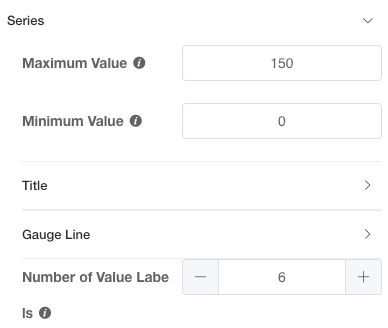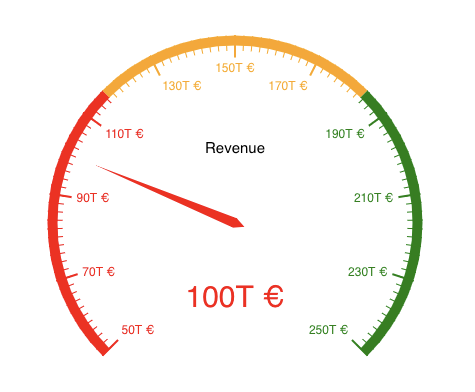# Introduction

The Gauge Chart displays one data point on a gauge:# Expected Data and Graph Designer

The Gauge Chart expects data in a two column format with the title (e.g. "Revenue") in the first column and the value in the second column:

NameAmount
Revenue100

The selection of the x-axis in the Graph Designer does not have an effect.

You can set the gauge's range (Maximum Value and Minimum Value) and the number of value labels shown on it  (Number of Value Labels) in the Graph Designer Series section:The values for the values labels are then automatically calculated.

By for example changing the values for the three fields as follows:The gauge chart will look like this:`As you might have noticed, the color of the gauge pointer changes according to the color of the range it is in.`

You can change the coloring option in SeriesGauge Line - Line Style and add sections by clicking the + button. Then you can choose how much of the gauge (in percent) the section should cover and which color it should have. The percentage value is the value until which it should be colored (i.e. the upper bound) and if there is another section with a lower percentage, this represents the lower bound: